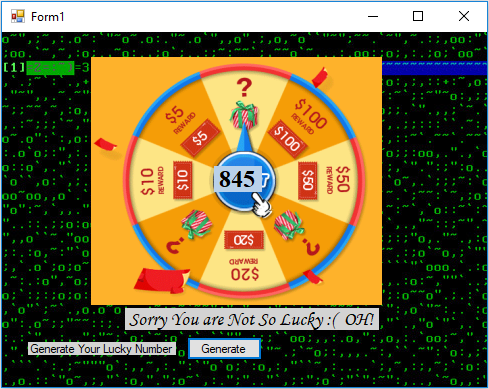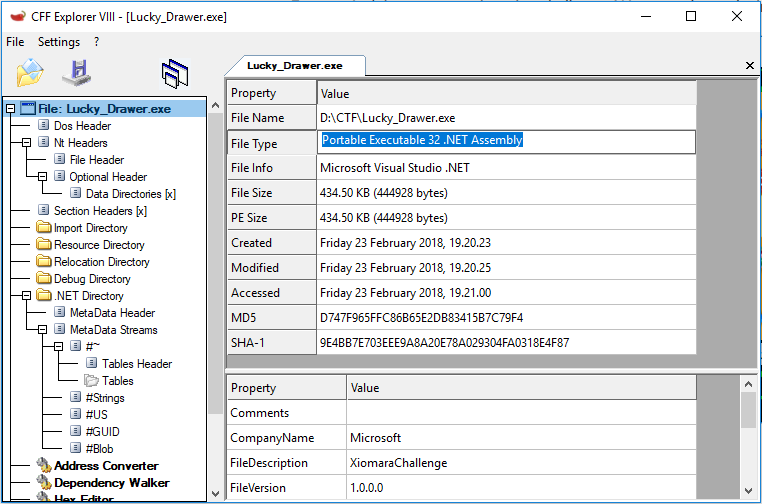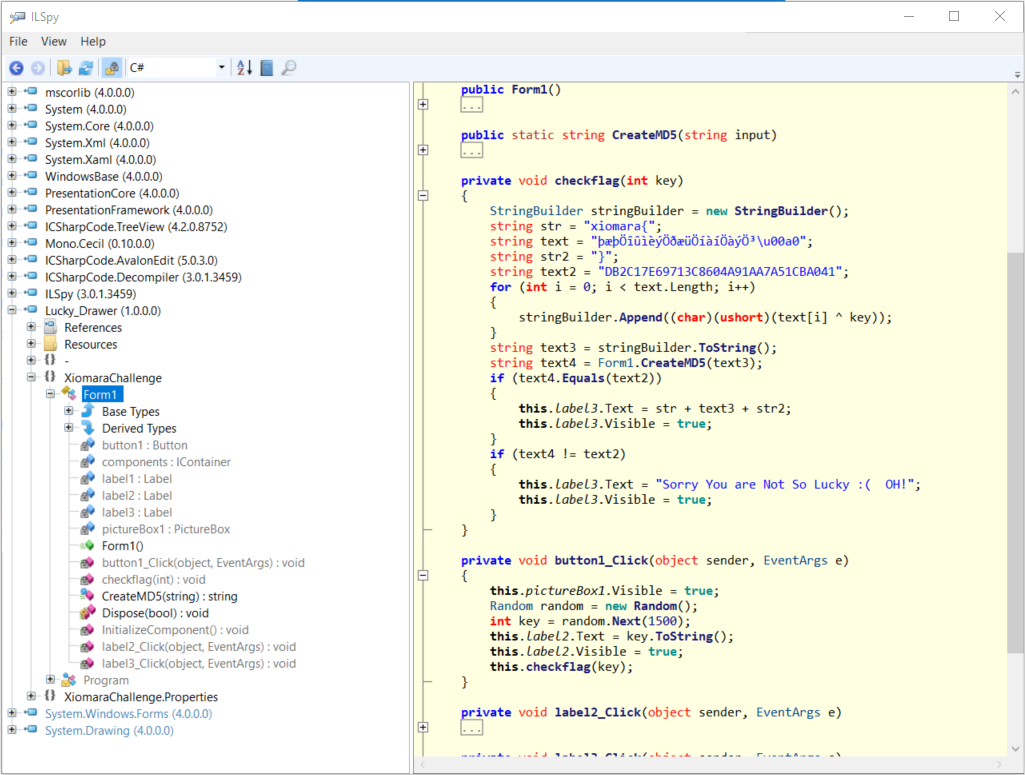# Fortune Jack

Xiomara CTF 2018 - RE (50 pts).

# Xiomara CTF 2018: Fortune Jack

Event Challenge Category Points Solves
Xiomara Fortune Jack Reverse 50 ¯\(ツ)

### Description

Fortune Jack is a reverse engineering challenge. We must play and win to get the flag.### TL;DR

The binary is a .NET executable. We can easily extract source code with ILSpy. The flag is ciphered with an XOR algorithm but key’s length is very small. I wrote a small python script which performs a brute force attack to recover the flag.

### Identification

I opened the binary with CFF Explorer. I looked the File Type field which indicates that binary is a “Portable Executable 32 bits .NET Assembly”.Now, we can choose the right tool for analysis.

### Analysis

I extracted binary’s source code with ILSpy Decompiler. As you will see below, the source code is not obfuscated, but the flag is ciphered.Look the algorithm, it’s a simple loop with a xor. The variable text will be deciphered by the key parameter which is an integer. In C# language, char represents a Unicode character. The value of a char is a 16 bits numeric value. This explains why the operation text[i] ^ key is cast into a ushort.

``````string str = "xiomara{";
string text = "þæþÖîûìèýÖðæüÖíàíÖàýÖ³\u00a0";
string str2 = "}";
string text2 = "DB2C17E69713C8604A91AA7A51CBA041";
for (int i = 0; i < text.Length; i++)
{
stringBuilder.Append((char)(ushort)(text[i] ^ key));
}
``````

If key is valid, md5 checksum of text (text4) will be equals to “DB2C17E69713C8604A91AA7A51CBA041” (text2)

``````string text3 = stringBuilder.ToString();
string text4 = Form1.CreateMD5(text3);
if (text4.Equals(text2))
{
this.label3.Text = str + text3 + str2;
this.label3.Visible = true;
}
``````

Key value is randomly generated between 0 and 1500.

``````Random random = new Random();
int key = random.Next(1500);
``````

### Scripting

I wrote a simple script which tries every key between 0 and 255 (because we want printable char). Script computes an md5 checksum of each result and checks if it’s equals to DB2C17E69713C8604A91AA7A51CBA041.

``````#-*- coding:utf-8 -*-
import hashlib
import binascii

# the ciphered flag in UTF-16
cipher = binascii.unhexlify("00FE00E600FE00D600EE00FB00EC00E800FD00D600F000E600FC00D600ED00E000ED00D600E000FD00D600B300A0")

def xor_md5(key):
s=""
for i in range(0,len(cipher),2):
value = (ord(cipher[i]) << 8) + ord(cipher[i+1]) # convert UTF-16 char into ushort
s+=chr(value ^ key) # xor with key
mD = hashlib.md5()
mD.update(s)
if mD.hexdigest().upper() == "DB2C17E69713C8604A91AA7A51CBA041":
print("xiomara{%s}" % s)

for i in range(0,255):
xor_md5(i)
``````

and the flag is …